### D.40 Quan­ti­za­tion of ra­di­a­tion de­riva­tions

This gives var­i­ous de­riva­tions for the ad­den­dum of the same name.

It is to be shown first that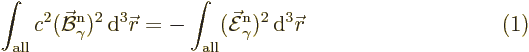To see that, note from (A.157) that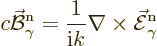so the left-hand in­te­gral be­comes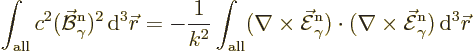Now the curl,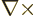, is Her­mit­ian, {D.10}, so the sec­ond curl can be pushed in front of the first curl. Then curl curl acts as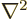be­cause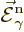is so­le­noidal and the stan­dard vec­tor iden­tity (D.1). And the eigen­value prob­lem turnsinto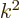.

Note in­ci­den­tally that the ad­di­tional sur­face in­te­gral in {D.10} is zero even for the pho­ton modes of def­i­nite an­gu­lar mo­men­tum, {A.21.7}, be­cause for them ei­theris zero on the sur­face or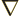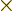is. Also note that the in­te­grals be­come equal in­stead of op­po­site if you push com­plex con­ju­gates on the first fac­tors in the in­te­grands.

Now the Hamil­ton­ian can be worked out. Us­ing Us­ing (A.152) and (A.162), it is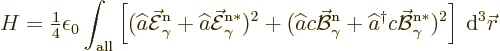When that is mul­ti­plied out and in­te­grated, the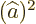and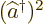terms drop out be­cause of (1). The re­main­ing mul­ti­plied-out terms in the Hamil­ton­ian pro­duce the stated Hamil­ton­ian af­ter not­ing the wave func­tion nor­mal­iza­tion (A.158).

The fi­nal is­sue is to iden­tify the re­la­tion­ships be­tween the co­ef­fi­cients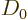,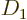and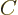as given in the text. The most im­por­tant ques­tion is here un­der what cir­cum­stances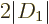and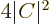can get very close to the larger value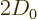.

The co­ef­fi­cientwas de­fined as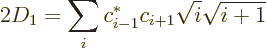To es­ti­mate this, con­sider the in­fi­nite-di­men­sion­al vec­tors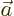and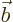with co­ef­fi­cients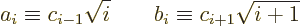Note that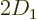above is the in­ner prod­uct of these two vec­tors. And an in­ner prod­uct is less in mag­ni­tude than the prod­uct of the lengths of the vec­tors in­volved.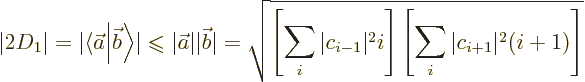By chang­ing the no­ta­tions for the sum­ma­tion in­dices, (let­ting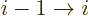and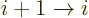), the sums be­come the ex­pec­ta­tion val­ues of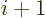, re­spec­tively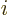. So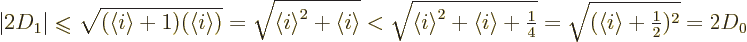The fi­nal equal­ity is by the de­f­i­n­i­tion of. The sec­ond in­equal­ity al­ready im­plies that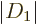is al­ways smaller than. How­ever, if the ex­pec­ta­tion value ofis large, it does not make much of a dif­fer­ence.

In that case, the big­ger prob­lem is the in­ner prod­uct be­tween the vec­torsand. Nor­mally it is smaller than the prod­uct of the lengths of the vec­tors. For it to be­come equal, the two vec­tors have to be pro­por­tional. The co­ef­fi­cients ofmust be some mul­ti­ple, call it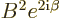, of those of: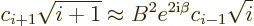For larger val­ues ofthe square roots are about the same. Then the above re­la­tion­ship re­quires an ex­po­nen­tial de­cay of the co­ef­fi­cients. For small val­ues of, ob­vi­ously the above re­la­tion can­not be sat­is­fied. The needed val­ues of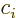for neg­a­tivedo not ex­ist. To re­duce the ef­fect of this start-up prob­lem, sig­nif­i­cant co­ef­fi­cients will have to ex­ist for a con­sid­er­able range ofval­ues.

In ad­di­tion to the above con­di­tions, the co­ef­fi­cienthas to be close to. Here the co­ef­fi­cientwas de­fined as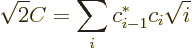Us­ing the same ma­nip­u­la­tions as for, but with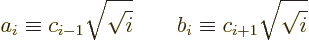gives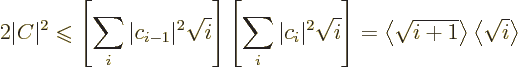To bound this fur­ther, de­fine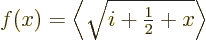By ex­pand­ing the square root in a Tay­lor se­ries,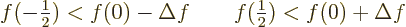where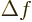is the ex­pec­ta­tion value of the lin­ear term in the Tay­lor se­ries; the in­equal­i­ties ex­press that a square root func­tion has a neg­a­tive sec­ond or­der de­riv­a­tive. Mul­ti­ply­ing these two ex­pres­sions shows that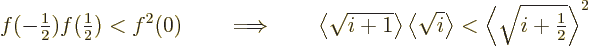Since it has al­ready been shown that the ex­pec­ta­tion value ofmust be large, this in­equal­ity will be al­most an equal­ity, any­way.

In any case,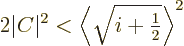This is less than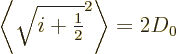The big ques­tion is now how much it is smaller. To an­swer that, use the short­hand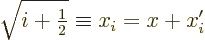where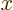is the ex­pec­ta­tion value of the square root and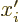is the de­vi­a­tion from the av­er­age. Then, not­ing that the ex­pec­ta­tion value ofis zero,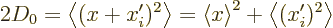The sec­ond-last term is the bound for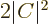as ob­tained above. So, the only way thatcan be close tois if the fi­nal term is rel­a­tively small. That means that the de­vi­a­tion from the ex­pec­ta­tion square root must be rel­a­tively small. So the co­ef­fi­cientscan only be sig­nif­i­cant in some lim­ited range around an av­er­age value of. In ad­di­tion, for the vec­torsandin the ear­lier es­ti­mate forto be al­most pro­por­tional,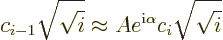where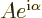is some con­stant. That again means an ex­po­nen­tial de­pen­dence, like for the con­di­tion on. Andwill have to be ap­prox­i­mately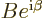. And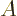will have to be about 1, be­cause oth­er­wise start and end ef­fects will dom­i­nate the ex­po­nen­tial part. That gives the sit­u­a­tion as de­scribed in the text.